### Effects Of Electric Current Class 10th Science And Technology Part 1 MHB Solution

Exercise

###### Exercise
Question 1.

Tell the odd one out. Give proper explanation.

a. Fuse wire, bad conductor, rubber gloves, generator.

b. Voltmeter, Ammeter, galvanometer, thermometer.

c. Loud speaker, microphone, electric motor, magnet.

(a) Odd one - bad conductor

Explanation: Fuse wire, rubber gloves, and generator are related to electric current. If a large amount of electric current flows it produce large amount of heat so fuse wire melts thus stopping the flow of current. Rubber gloves do not conduct heat or electricity which we wear while handling electrical equipment to protect from shocks.

The generator is based on magnetic effects of electric current which converts mechanical energy into electrical energy.

But bad conductor is Not related to electric current.

(b) Odd- Thermometer.

Explanation: Thermometer measures heat. But ammeter, voltmeter and galvanometer measures electric current, potential difference, a small amount of current respectively in an electrical circuit.

(c) Odd- magnet

Explanation: Loudspeaker, microphone and electric motor works on principles of some effects of electric current.

But Magnet has magnetic properties which attracts magnetic substances towards it and has no relation with electric current.

Question 2.

Explain the construction and working of the following. Draw a neat diagram and label it.

Electric motor

Electric motor- It converts electrical energy into mechanical energy.

Principle- When a current carrying conductor is placed normally in a magnetic field it experiences a force which rotates the conductor thus mechanical energy is generated.

Construction-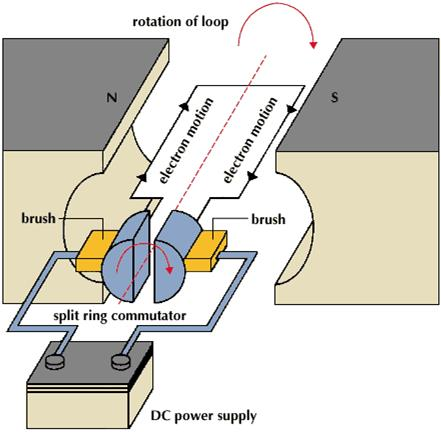It consists of an armature coil ABCD mounted on an axle. It also consists of two split rings P and Q with brushes X and Y and a horse-shoe electromagnet NS with a dc source.

The rectangular coil is wound around a soft iron core and is placed between two poles of horse-shoe electromagnet. The coil is free to rotate about its axis. The ends A and D are connected to two split rings P and Q which are attached with brushes X and Y respectively. When coil rotates split rings also rotates but brushes remain fixed at their position.

Working-

i. When current enters the coil ABCD from battery through brush X and to coil and finally back to brush Y the current flow in coil from A to B upwards and C to D downwards.

ii. So on applying Fleming’s left-hand rule, a force is exerted on arm AB which pushes it downwards and forces on arm CD upwards thus rotating the coil anticlockwise. As both forces are equal and opposite in direction rotating the coil.

iii. After half rotation split, ring P contact with brush Y and split ring Q contact with brush X thus current will flow in DCBA path.

iv. Now force acting on both arms of the coil will be reversed and coil will continue to rotate according to Fleming’s left-hand rule and complete the next half turn in the same direction. Thus the coil rotates continuously.

Question 3.

Explain the construction and working of the following. Draw a neat diagram and label it.

Electric Generator (AC)

Electric generator AC converts mechanical energy into electrical energy.

Principle- It works on the principle of electromagnetic induction. When coil rotates in a magnetic field it induces a current in the coil and direction of induced current is given by Fleming’s right hand rule.

Construction-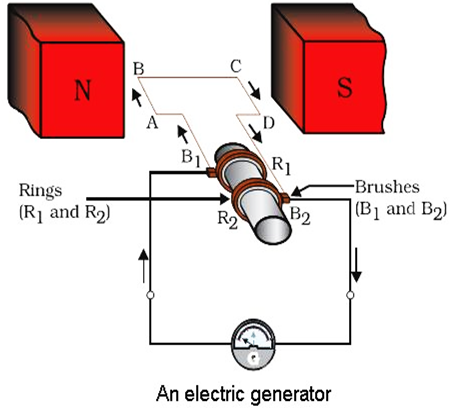It consists of a coil ABCD with two slip rings R1 and R2 connected with brushes B1 and B2 respectively. The coil is placed between two poles of a horse-shoe shaped electromagnet. Brushes are connected to a galvanometer which shows the direction of current in the circuit.

Working- When coil rotates the arm AB goes up and arm CD goes downwards, so coil rotates in clockwise direction. Applying Fleming’s right hand rule the induced current which is produced is in the direction ABCD. Current flows from brush B2 to B1. After half rotation AB comes in place of CD and CD in place of AB thus induced current flows from DCBA. Now current flow from brush B1 to B2. Thus every half rotation current flow direction is reversed thus it is an alternating current.

Question 4.

Electromagnetic induction means

a. Charging of an electric conductor.

b. Production of magnetic field due to a current flowing through a coil.

c. Generation of a current in a coil due to relative motion between the coil and the magnet.

d. Motion of the coil around the axle in an electric motor.

C

When there is relative motion between coil and a magnet there is change in magnetic field lines which induces a current in the coil. This phenomenon is called electromagnetic induction discovered by Michael Faraday.

Question 5.

Explain the difference:

AC generator and DC generator.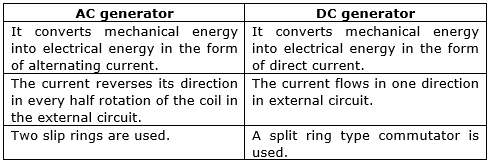Question 6.

Which device is used to produce electricity? Describe with a neat diagram.

a. Electric motor

b. Galvanometer

c. Electric Generator (DC)

d. Voltmeter

C

It converts mechanical energy into electrical energy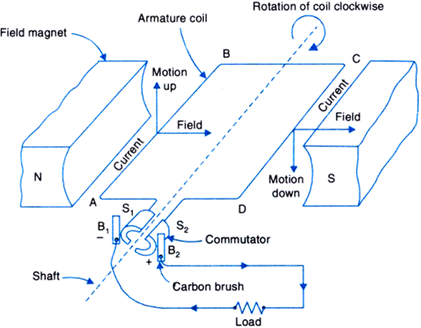It consists of armature coil ABCD which is placed between two poles of a horse-shoe shaped electromagnet, two split rings S1 and S2 connected to two brushes B1 and B2 respectively.

When the coil is rotated the magnetic lines changes thus induces a current in the coil. The direction of induced current in the arms of coil reverses after every half rotation which can be obtained by Fleming’s right-hand rule. Here one brush is always in contact with the arm moving up in the field and another brush is in contact with the arm moving downward. So, a unidirectional current flows in the circuit.

Question 7.

How does the short circuit form? What is its effect?

When live wire comes in contact with neutral wire a large amount of current flows through it leading to short-circuiting. The resistance of circuit becomes very small thus a large number of current flows leading to the production of a large amount of heat thus may cause electric fires. This may damage our electrical appliances. We can also get electric shocks thus short-circuiting is dangerous.

Question 8.

Give Scientific reasons.

Tungsten metal is used to make a solenoid type coil in an electric bulb.

Tungsten is a strong metal with the high melting point of 3380oC. When bulb glows a large amount of heat is produced and the temperature is also very high. So tungsten is used which can resist this huge amount of heat and high temperature.

Question 9.

Give Scientific reasons.

In the electric equipment producing heat e.g. iron, electric heater, boiler, toaster etc, an alloy such as Nichrome is used, not pure metals.

Resistivity of alloys like nichrome is more than pure metals so they will get heated easily on the passage of a small amount of current. They also have a high melting point and do not oxidize readily at high temperature. So in the electric equipment producing heat e.g. iron, electric heater, boiler, toaster etc, an alloy such as Nichrome is used, not pure metals.

Question 10.

Give Scientific reasons.

For electric power transmission, copper or aluminum wire is used.

Copper or aluminum are metals which have free electrons so they are good conductors of electricity. They have a low value of resistivity and are cheap and readily available so for electric power transmission, copper or aluminum wire is used.

Question 11.

Give Scientific reasons.

In practice, the unit kWh is used for the measurement of electrical energy, rather than joule.

As joule is a very small unit of energy but kWh is a large unit of measuring electrical energy so in practice the unit kWh is used for the measurement of electrical energy, rather than joule.

1 kWh = 3.6 × 106 J

Question 12.

Which of the statement given below correctly describes the magnetic field near a long, straight current-carrying conductor?

a. The magnetic lines of force are in a plane, perpendicular to the conductor in the form of straight lines.

b. The magnetic lines of force are parallel to the conductor on all the sides of the conductor.

c. The magnetic lines of force are perpendicular to the conductor going radially outward.

d. The magnetic lines of force are in concentric circles with the wire as the center, in a plane perpendicular to the conductor.

d

When current is passed through a long, straight current-carrying wire a magnetic field is developed where magnetic field lines are in form of concentric circles around the wire in the center with their plane perpendicular to the straight wire. We can obtain these magnetic lines of force by sprinkling some iron fillings in a cardboard with a straight long wire passing through the center of the cardboard. This arrangement is connected to battery, key, rheostat, and ammeter. When current will pass through this iron fillings will get arranged in form of concentric circles around the wire.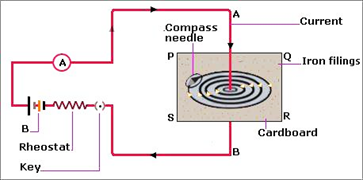Question 13.

What is a solenoid? Compare the magnetic field produced by a solenoid with the magnetic field of a bar magnet. Draw neat figures and name various components.

A solenoid is a coil of many circular turns of insulated copper wire wrapped closely in the shape of a cylinder.

Similarities-

i. The properties of the magnetic field produced due to a solenoid are similar to the properties of the magnetic field produced by a bar magnet.

ii. The magnetic field lines are closed continuous curves.

iii. They emerge from North pole and ends at South pole.

iv. No two magnetic lines intersect.

v. Both have magnetic properties and they attract magnetic materials towards them.

Dissimilarities-

i. The strength of magnetic field produced by a solenoid can be increased by increasing the amount of current which cannot be done in case of a bar magnet.

ii. The direction of the magnetic field can be changed by reversing the current in a solenoid but it cannot be done in case of a bar magnet.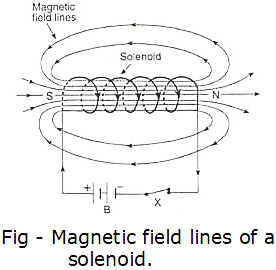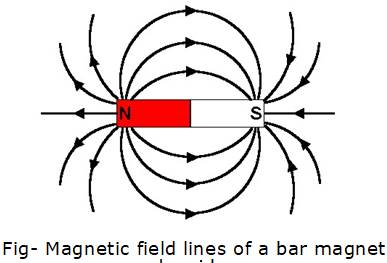Question 14.

Name the following diagrams and explain the concept behind them.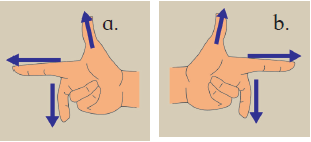Figure a is Fleming’s Right-hand rule which is used to find out the direction of induced current. The thumb, forefinger and central finger of right hand is stretched in such a way that these three fingers are mutually perpendicular to each other. If forefinger indicates the direction of magnetic field, thumb indicates the direction of motion of conductor then central finger will indicate the direction of induced current.

Figure b is Fleming’s left-hand rule which is used to find the direction of motion of conductor. The thumb, forefinger and central finger of the left hand is stretched in such a way that these three fingers are mutually perpendicular to each other. If forefinger indicates the direction of magnetic field, central finger indicates the direction of current then thumb will indicate the direction of motion of conductor.

Question 15.

Identify the figures and explain their use.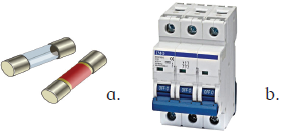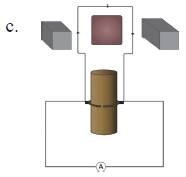(i) a is cartridge type fuse which is fitted with costly appliances like refrigerator, AC, geyser, TV etc. They have a proper current rating like 1 A, 2 A, 5 A etc. If the amount of current flow exceeds their ratings the fuse melts thus the circuit opens and current flow stops protecting the appliance from damage during overloading, short-circuiting or faulty in appliances.

(ii) b is MCB[ miniature circuit breakers ] is automatically operated switch. When current flow exceeds their limit during short-circuiting or overloading they automatically trip down thus breaking the circuit and stops the flow of current. Thus protects the electrical appliances from damage and also from getting electrical shocks. They are mainly used in low voltage electrical network.

(iii) c is an electric motor which converts electrical energy into mechanical energy. It is used in electric gadgets like a fan, juicer, washing machine, mixer, grinder etc.

Question 16.

Solve the example.

Heat energy is being produced in a resistance in a circuit at the rate of 100 W. The current of 3 A is flowing in the circuit. What must be the value of the resistance?

Answer: Given Power P = 100 W

Current I = 3 A

R =?

We know P = I2R;

where R is resistance

So R = P/ I2

⇒, R =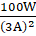= 11.11 ohm

Question 17.

Solve the example.

Two tungsten bulbs of wattage 100 W and 60 W power work on 220 V potential difference. If they are connected in parallel, how much current will flow in the main conductor?

We know P = VI

Where P = power

V = potential difference

I = current

Here V = 220 V

P = 100 W + 60 W = 160 W

[ Since bulbs are connected in parallel ]

I =?

I = P/V

= 160W/220V

I = 0.72 A

Question 18.

Solve the example.

Who will spend more electrical energy? 500 W TV Set in 30 mins, or 600 W heater in 20 mins?

We know Energy spent = P x t

Where P = power

t = time

in case of tv set

P = 500 W

t = 30 minutes = 1/2 hour

so, energy spent = 500 x 1/2

= 250 Wh

In case of heater

P = 600 W

t = 20 minutes = 0.33 hour

so, energy spent = 600 x 0.33

= 198 Wh

Hence TV set spent more electrical energy.

Question 19.

Solve the example.

An electric iron of 1100 W is operated for 2 hrs daily. What will be the electrical consumption expenses for that in the month of

April? (The electric company charges Rs 5 per unit of energy).

Power of electric iron = 1100 W

Time t = 2 hrs

So energy consumed daily = P x t

= 1100 x 2

= 2200 Wh

For 30 days since the month of april has 30 days

Energy consumed= 2200 x 30

= 66000 Wh

= 66 Kwh

1 Kwh = 1 unit = Rs 5

So 6.6 Kwh = 5 x 66 = 330 rupees

So the bill for month of April will be 330 rupees.

## PDF FILE TO YOUR EMAIL IMMEDIATELY PURCHASE NOTES & PAPER SOLUTION. @ Rs. 50/- each (GST extra)

SUBJECTS

HINDI ENTIRE PAPER SOLUTION

MARATHI PAPER SOLUTION
SSC MATHS I PAPER SOLUTION
SSC MATHS II PAPER SOLUTION
SSC SCIENCE I PAPER SOLUTION
SSC SCIENCE II PAPER SOLUTION
SSC ENGLISH PAPER SOLUTION
SSC & HSC ENGLISH WRITING SKILL
HSC ACCOUNTS NOTES
HSC OCM NOTES
HSC ECONOMICS NOTES
HSC SECRETARIAL PRACTICE NOTES

2019 Board Paper Solution

HSC ENGLISH SET A 2019 21st February, 2019

HSC ENGLISH SET B 2019 21st February, 2019

HSC ENGLISH SET C 2019 21st February, 2019

HSC ENGLISH SET D 2019 21st February, 2019

SECRETARIAL PRACTICE (S.P) 2019 25th February, 2019

HSC XII PHYSICS 2019 25th February, 2019

CHEMISTRY XII HSC SOLUTION 27th, February, 2019

OCM PAPER SOLUTION 2019 27th, February, 2019

HSC MATHS PAPER SOLUTION COMMERCE, 2nd March, 2019

HSC MATHS PAPER SOLUTION SCIENCE 2nd, March, 2019

SSC ENGLISH STD 10 5TH MARCH, 2019.

HSC XII ACCOUNTS 2019 6th March, 2019

HSC XII BIOLOGY 2019 6TH March, 2019

HSC XII ECONOMICS 9Th March 2019

SSC Maths I March 2019 Solution 10th Standard11th, March, 2019

SSC MATHS II MARCH 2019 SOLUTION 10TH STD.13th March, 2019

SSC SCIENCE I MARCH 2019 SOLUTION 10TH STD. 15th March, 2019.

SSC SCIENCE II MARCH 2019 SOLUTION 10TH STD. 18th March, 2019.

SSC SOCIAL SCIENCE I MARCH 2019 SOLUTION20th March, 2019

SSC SOCIAL SCIENCE II MARCH 2019 SOLUTION, 22nd March, 2019

XII CBSE - BOARD - MARCH - 2019 ENGLISH - QP + SOLUTIONS, 2nd March, 2019

HSC Maharashtra Board Papers 2020

(Std 12th English Medium)

HSC ECONOMICS MARCH 2020

HSC OCM MARCH 2020

HSC ACCOUNTS MARCH 2020

HSC S.P. MARCH 2020

HSC ENGLISH MARCH 2020

HSC HINDI MARCH 2020

HSC MARATHI MARCH 2020

HSC MATHS MARCH 2020

SSC Maharashtra Board Papers 2020

(Std 10th English Medium)

English MARCH 2020

HindI MARCH 2020

Hindi (Composite) MARCH 2020

Marathi MARCH 2020

Mathematics (Paper 1) MARCH 2020

Mathematics (Paper 2) MARCH 2020

Sanskrit MARCH 2020

Important-formula

THANKS